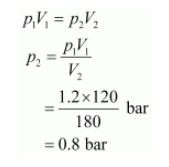# A vessel of 120 mL capacity contains a certain amount of gas at 35 °C and 1.2 bar pressure

Question:

A vessel of 120 mL capacity contains a certain amount of gas at 35 °C and 1.2 bar pressure. The gas is transferred to another vessel of volume 180 mL at 35 °C. What would be its pressure?

Solution:

Given,

Initial pressure, p1 = 1.2 bar

Initial volume, V= 120 mL

Final volume, V2 = 180 mL

Since the temperature remains constant, the final pressure (p2) can be calculated using Boyle’s law.

According to Boyle’s law,Therefore, the pressure would be 0.8 bar.